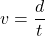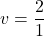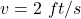## Alessandra jumps on a trampoline. It takes her 1 second to reach a height of 2 feet above the trampoline. What is the speed of A

Question

Alessandra jumps on a trampoline. It takes her 1 second to
reach a height of 2 feet above the trampoline.
What is the speed of Alessandra’s motion?
A. 2 ft/s
B. 0.5 ft/s
C. 2 ft/s up
D. 0.5 ft/s down

in progress 0
3 months 2021-07-22T00:35:06+00:00 2 Answers 21 views 0

The speed of Alessandra’s motion is 2 ft/s up.

(C) is correct option.

Explanation:

Given that,

Time = 1 sec

Height = 2 feet

We need to calculate the speed of Alessandra’s motion

Using formula of speedWhere, v = speed

d = height

t = time

Put the value into the formulaThe direction will be up ward.

Hence, The speed of Alessandra’s motion is 2 ft/s up.

(C) is correct option.